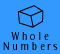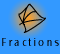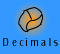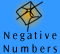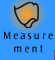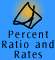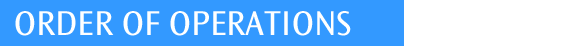| Why do we need it? | How to use brackets | The basic rules | The complete rules | Using calculators | Quick quiz |

Why do we need an order of operations?

Example: In a room there are 2 teacher's chairs and 3 tables each with 4 chairs for the students. How many chairs are in the room?

We know there are 14, but how do we write this calculation? If we just write
2 + 3 x 4

2 + 3 = 5, then multiply by 4 to get 20 or
3 x 4 = 12, then 2 + 12 to get 14?
There are two steps needed to find the answer; addition and multiplication. Without an agreed upon order of when we perform each of these operations to calculate a written expression, we could get two different answers. If we want to all get the same "correct" answer when we only have the written expression to guide us, it is important that we all interpret the expression the same way.

One way of explaining the order is to use brackets. This always works. To say that the 3 x 4 is done before the adding, we would use brackets like this:
2 + (3 x 4)
The brackets show us that 3 x 4 needs to be worked out first and then added to 2. However, we can also agree on an order of operations, which is explained below.

Another example: Calculate 15- 10 ÷ 5

If you do the subtraction first, you will get 1. If you do the division first, which is actually correct according to the rules explained below, you will get 13. We need an agreed order.

 division first (correct) subtraction first blue indicates the operations being worked on first 15 - 10 ÷ 5 15 - 10 ÷ 5 = 15 - 10 ÷ 5 = 15 - 10 ÷ 5 = 15 - 2 = 13 = 5 ÷ 5 = 1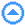How to use brackets

Brackets are marks of inclusion which tell us which parts of an expression go together. We use brackets in an expression to indicate which part to calculate first. It can be useful to think of brackets as a circle with the top and bottom deleted to remind you that brackets indicate that everything inside the 'circle' is self-contained and must be worked out first. Although brackets usually look like ( ), brackets can also look like { } or [ ] and need to be treated in the same way. Brackets are sometimes referred to as "parentheses".

 want division first want subtraction first blue indicates the operations being worked on first 15 - (10 ÷ 5) (15 - 10) ÷ 5 = 15 - (10 ÷ 5) = (15 - 10) ÷ 5 = 15 - 2 = 13 = 5 ÷ 5 = 1

There are more examples on how to use brackets in complicated examples below.

If we used brackets consistently we would not have to be concerned with the order of operations. We could just work from innermost brackets outwards to eventually get our answer. However using lots of brackets can become tedious and confusing, as in the following example, so we need some agreed rules.

 3 + ((4÷2)x7)-(6÷3)-((4x2)+((8÷2)+(3x3)))

You can check how to work out this monster by clicking here, but the next section tells you how to avoid the worst monsters.

YOU CAN ALWAYS USE BRACKETS TO SHOW HOW
A CALCULATION SHOULD BE DONEThe basic rules

Many years ago mathematicians decided on an 'order of operations' that everyone should use when performing mathematical computations from written instructions. This means that when presented by the same problem everyone using this agreed convention of order of operations would obtain the same answer. You could think of the order of operations as a sort of 'maths grammar' which enables mathematicians to communicate with each other and with machines all over the world.

It is important to realise that the order of operations has nothing to do with underlying mathematical principles: it is just convention. Other rules could have been invented. However the convention needs to be understood before it can be successfully applied to every problem.

The four rules below are enough for most purposes:

 • RULE 1: Calculate anything in brackets first, then apply the other rules. (For further discussion about expressions with more than one set of brackets, see the next section.) • RULE 2: If a calculation involves only addition and subtraction, work from left to right. • RULE 3: If a calculation involves only multiplication and division, work from left to right. • RULE 4: Do multiplication and division before addition and subtraction.

Example of Rule 2: 10 - 3 + 2
This involves only addition and subtraction, so we work from left to right. 10 - 3 + 2 is equal to 9 because we calculate 10 - 3 first, then add 2. We do NOT do 3+2 first, then subtract from 10.

Example of Rule 3: 48 ÷ 2 x 3
This involves only multiplication and division, so we work from left to right. 48÷ 2 x 3 is equal to 72, because 48 ÷ 2 = 24 and 24 x 3 = 72.
We do NOT work out 2 x 3 = 6 and then do 48 ÷ 6 = 8.

Example of Rule 4: 4 + 5 x 3
Multiplication has precedence over addition. 4 + 5 x 3 is equal to 19 because 5 x 3 = 15 and 4 + 15 is 19. We do NOT work out 4 + 5 first to get 9 and then multiply by 3.

Example of Rule 1: (4 + 5) x 3
(4 + 5) x 3 is equal to 27, because we calculate the brackets first to 4 + 5 = 9 and then multiply by 3. We do NOT work out 5 x 3 and then add 4.

Examples using all of the rules together:

Example: 72 + 4 x 6 ÷ 2 - 8

Working out Thinking text
 72 + 4 x 6 ÷ 2 - 8 We have addition, multiplication, division and subtraction in this expression. = 72 + 4 x 6 ÷ 2 - 8 Using RULE 4 = 72 + 4 x 6 ÷ 2 - 8 Using RULE 3 = 72 + 24 ÷ 2 - 8 Using RULE 3 = 72 + 12 - 8 Using RULE 2 = 84 - 8 Using RULE 2 = 76

Example: 15 - 12 ÷ (6 ÷ 2) x 4

Working out Thinking text
 15 - 12 ÷ (6 ÷ 2) x 4 + 3 We can see that we have brackets, multiplication and division and addition and subtraction in this expression. = 15 - 12 ÷ (6 ÷ 2) x 4 + 3 First, the brackets. (Rule 1) = 15 - 12 ÷ 3 x 4 + 3 Then division and multiplication, working from left to right. (Rules 4 and 3) = 15 - 4 x 4 + 3 = 15 - 16 + 3 Then addition and subtraction, working from left to right. (Rule 2) = - 1 + 3 = 2The complete rules

BODMAS, BOMDAS, BEMDAS, BIDMAS etc..

Many of us were taught to use the BODMAS or BOMDAS mnemonics or other variations to determine the order of operations. They summarise the rules above:
brackets first,
then multiplication or division (left to right)
then addition or subtraction(left to right).

 Brackets Either BODMAS or BOMDAS must be interpreted as implying the same order of operations. then Of or Multiplication or Division then Addition or Subtraction

However blind adherence to these mnemonics (memory aides) without understanding of the mathematical ideas they represent will lead to misunderstandings and incorrect usage, particularly when they are applied to more complicated expressions.

B O D M A S - the "B"

"B" comes first, so in evaluating an expression, do the brackets first. We have talked about how and why brackets are used in the section above, How to use brackets. But what if there are several brackets?

 Rule for multiple brackets: If there are brackets in the expression, calculate them first. If there is more than one set of brackets then begin with the innermost brackets and work outwards. If there is more than one set of brackets but they are isolated from each other, then do them independently.

Example: Expressions with multiple brackets.
3 x ((2+(3x4)) + (5(8÷4) - 9))

 Working Out ThinkingWe work on the innermost brackets first. Here there are 2 isolated sets of inner brackets. Then we move to the next level of brackets and so on.

B O M D A S - the "O"

The 'O' in BODMAS stands for 'of', which is a verbal indication of multiplication. It is really included as a convenient vowel for the mnemonic to work as a word.

 Did you know that in other versions of the memory aide, such as BIDMAS and BEDMAS, the 'O' has been replaced by 'I' for indicies or 'E' for exponents respectively. This is useful as it extends the mnemonic to expressions which involve squares etc. See below.

B O M D A S or B O D M A S - the "M" and the "D"

Common misconception 1: BOMDAS tells me to do multiplication before division.
Common misconception 2: BODMAS tells me to do division before multiplication.

 Actual rule: Multiplication and division are inverse operations and as such need to be treated equally. When confronted with multiplication and division, always work from left to right.

Example: 105 ÷ 3 x 5
105 ÷ 3 x 5
is equal to 175 because we work out 105 ÷ 3 = 35 first and then multiply by 5. We do NOT work out 3 x 5 = 15 first and then divide 105 by 15. This would give us an incorrect answer of 7. BOMDAS and BODMAS give the same answer, correctly interpreted.

B O M D A S or BODMAS - the "A" and the "S"

Common misconception 3: BOMDAS or BODMAS tells me to do addition before subtraction.
Common misconception 4: It doesn't matter what order you do addition or subtraction.

 Actual rule: Addition and subtraction are inverse operations and as such need to be treated equally. When confronted with addition and subtraction, always work from left to right.

Example: 3 + 7 - 4 - 9
3 + 7 - 4 - 9 is equal to - 3 because we work out the addition first, 3 + 7 = 10, and 10 - 4 = 6, then 6 - 9 = - 3.

We can see that if we did NOT work from left to right and worked out 4 - 9 = - 5 first, and then subtracted this from 3 + 7 then,
3 + 7 - (- 5) = 10 + 5 = 15

B I D M A S OR B E D M A S - the "I" or "E"

Powers, fractions and roots

Powers (also known as exponents or indicies), fractions and roots are not covered by BODMAS or BOMDAS but we still need to know how to handle them. Fractions, powers, roots and other self contained parts of expressions should be treated as if they are in brackets, i.e. work them out first.

Example: 4 + 2 3 x 6
We treat 2 3 as if it is in brackets and work this out first. 2 3 is equal to 8. Then we continue with, 4 + 8 x 6 which is equal to 52 because we do the multiplication first and then the addition.

Example: (2 + 3) 2
In this expression the brackets around the addition of 2 + 3 indicate that it is 2 + 3 that is raised to the power of 2, NOT just 3. We must work out the brackets first and then square the answer. 5 2 = 10

Example: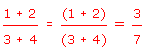Actual rule: Fractions should be treated as if the numerator is in brackets and the denominator is in brackets and the fraction bar (the "vinculum") is division.

Example: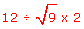We work out the square root first to get 3 and then do the division and multiplication working from left to right. 12 ÷ 3 x 2 is equal to 8.

We cannot do anything withuntil it has been simplified. Also, you will notice once again that if we do the multiplication before the division then we will get a different answer.

What to remember:

Work on one level at a time, starting at the top and going down.

Within each level, work from left to right.

 Brackets powers, roots and fractions multiplication and division addition and subtractionActivities

Four fours

Using four fours and any mathematical operations and signs you wish, can you make every number from 1 to 20. Can you make every number up to 100?

For example, (4 +4) x 4 - 4 = 28 and 4 + (4 x 4) - 4 = 16.

This is an excellent activity for a class to do over a week. Make a large chart with a space for one or more expressions for each number. Students can enter their expressions on the class chart after they have been checked. The teacher can decide what signs are allowed.

Manipulating expressions

6 + 17 - 15 x 4 ÷ 3

By inserting brackets into this expression (as many as you like, wherever you like) make expressions with as many answers as you can.

The correct answer when there are no brackets is
6 + 17 - 15 x 4 ÷ 3 = 3.

This set of inserted brackets changes the answer to 8.66:
6 + (17 - 15) x 4 ÷ 3 = 6 + 2 x 4 ÷ 3 = 6 + 8 ÷ 3 = 6 + 2.66 = 8.66Using calculators

Not all calculators have correct order of operations built in. More sophisticated calculators have programmed logic which enables them to use the standard mathematical conventions. Others just process the information/keystrokes exactly as they are entered.

Example: If you need to calculate 1 + 5 x 7 and enter these 6 key presses:

 1 + 5 x 7 =

some calculators give 42 (1 + 5 gives 6, multiply by 7 gives 42) and others give 36 (multiply first so 5 x 7 = 35, add 1 + 35 giving 36).
The second is the correct answer for the expression.

Find out how your calculator works and check to see if it has brackets to help be precise. Learn how to use the memory to keep intermediate answers.Quick quiz

 1. Using the example 10 - 1 - 2 , show why you need to follow the correct order of operations. 2. Calculate the following expressions: (a) 11 x (3 + 2) x 4 ÷ 2 (b) 7 - 18 ÷ 2 x 3 + 5 (c) 42 ÷ 3 x 7 3. Calculate 9 + 4 ÷ 2 x 7 - 6 ÷ 3 - 4 x 2 + 8 ÷ 2 + 3 x 3 4. Using the expression in question 3, make 3 alternative expressions and answers by inserting brackets. 5. Find the answer to,showing the method you have used to ensure you follow the correct order of operations, e.g. checklist, colour scheme, arrows etc. 6. Calculate the following expressions: (a) 32 ÷ 42 x (3 - 8) (b) 81 ÷ (4 - 7)3 (c)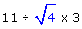(d)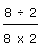(e)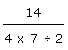7. Find out how to use your calculator to evaluate the expressions in question 6. 8. Bernie is in the process of landscaping the gardens of two new townhouses. If he buys 30 bundles of 12 wooden planks for the fence for each house and 15 bundles of 10 hardwood planks for the decking for each house, write an expression for the total number of planks bought and then work it out. If Bernie then returned 2 bundles of the wooden fence planks but bought 5 extra bundles of the hardwood planks, write a new expression and then work out the answer. 9. Two thirds of all Year 8 students, one quarter of all Year 9 students, only 30 Year 10 students and two fifths of Year 11 and 12 students combined ride their bike to school. If there are 99 Year 8 students, 124 Year 9 students, 111 Year 10 students, 65 Year 11 students and 50 Year 12 students attending the school, how many students ride their bike to school. Write a mathematical expression for the number of students who ride to school and then find the answer. 10. (300 ÷ (10 x 2)) x 4. Create an appropriate worded problem from this mathematical expression.Monster multiple brackets example!

 3 + ((4÷2)x7) - (6÷3) - ((4x2) + ((8÷2) + (3x3))) = 3 + ((4÷2)x7) - (6÷3) - ((4x2) + ((8÷2) + (3x3))) = 3 + ((2)x7)) - (2) - ((8) + ((4) + (9)) = 3 + (14) - (2) - (8 + (13)) = 3 + (14) - (2) - (21) = 3 + 14 - 2 - 21 = 17 - 2 - 21 = 15 - 21 = -6